Elementary Structures of MatterAuthor: Burkhard HEIM Subject: Physics, relativity, unified field theory, elementary particles, matter, Abstract: A brief introduction into the very complex work of Burkhard Heim is given. Copyright: Copyright for Contents by Burkhard Heim, Northeim, 1998; Translation by Prof. Dr. Hans Auerbach, Gebenstorf, 1998. Copyright for HTML-Design: Bernhard Harrer, Berlin, 1999 Info Jockey's Comment: This article has also been published in german language. It is not yet easy to convert a text like this in HTML-format. Nevertheless I am very glad to present you this english translation of an article by Burkhard Heim. [IJBH]Elementary Structures of Matter

In physics a number of approaches are possible in order to try to arrive at a comprehensive, unified theory describing a maximum number of observable phenomena.

One such possibility would be the geometrization of physical structures described in the two volumes Elementarstrukturen der Materie (Elementary Structures of Matter)  and . A clear advantage of this approach is the fact that space and object are no longer foreign to each other. Instead, an object now appears as a specific metric structure of space. A consequence of this is the unity of field and field source. The first attempt at such a geometrization, applied to the gravitational field, was carried out by A. Einstein in his general theory of relativity, later on extended by Kaluza, Klein, and Penrose, but also by P. Jordan and others.

The theory of supergravity and superstring theory may be regarded as successors to the KaluzaKlein model. At present, superstring theory is being further developed in the hope of attaining a unified description in a 10dimensional space, R10 , of the 4 empirically known interactions. However, it is not clear how the predictions of this theory, involving particle masses of some 1018 GeV, can be verified in view of the limitations of presentday high energy experiments. Here a radical geometrization of space as described in  and  appears to lead to more suitable results that may readily be compared with observation.

An entirely different approach to the unified description of nature by the use of group theoretical arguments has been applied with some success to the unification of the 4 known elementary forces. So far it has not also been possible to verify these theories experimentally, again since the resulting mass spectrum of elementary particles involves much too high energies. In addition, there is a lack of predictions concerning quantum numbers of resonance spectra representing these masses and about their upper limits.

By extending the ideas of Einstein, Kaluza, Klein and Jordan the theory outlined in this report shows how to geometrize in principle not only the gravitational field but the other force fields as well. They appear as geometrical structures of spacetime, R4 , (a Minkowski space with x4 = ict) subject to the usual conservation laws, and lead to a general nonHermitian geometry in R4 . The covariant components of the corresponding triple index symbols,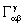, representing generalized Christoffel symbols, can be split into a Hermitian and an antiHermitian part, but, in contrast to Riemannian geometry, they cannot in general be expressed explicitly in terms of derivatives of the fundamental metric tensor, unless additional conditions are introduced. However, since such conditions reduce the generality it is not known whether they are at all admissible from a physical point of view. Thus, thesymbols are to be treated as components of a unified field of metric structures.

A transition to the microscopic region by use of the correspondence principle turns the unified field components into true tensor components with respect to the group of onetoone, continuous, and nonsingular coordinate transformations (Poincare´group), with mixed covariant and contravariant indices. This also applies to the macroscopic domain, where a unique set of metric structures corresponds to each type of field (gravitational, e.m.). Different fields, characterized by different conservation laws, lead to different geodetic conditions.

On passing over to the microscopic domain thesymbols, which are non Hermitian in their covariant indices, transform into components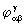of a tensor field with mixed indices. The condition= 0 can only be satisfied if the corresponding R4 is completely unstructured. In the microscopic range the phenomenological energy density leads to a discrete set of eigenvalues and eigenfunctions,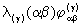, characterizing a discrete spectrum of metric structures (parantheses around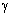denote suspension of the summation convention). The concept of energy density appears in geometrized form because phenomenological energies are time derivatives of actions, so that spatial energy densities ultimately are spacetime dependent action densities. Hence, due to the quantization of actions it is impossible in the limit to make the transition to differential quotients. This implies the necessary existence of a smallest geometric unit.

The nonlinear and nonHermitian equations of state satisfied by the R4 structuresexhibit algebraic symmetries such that 28 out of the total of 43 = 64 relations for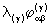always remain empty. Thus, 36 equations lead to relations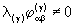, while the empty level spectra of geometrical structures with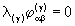require thatbecause. The fact that geometrical structures in the microscopic range vary in discrete steps is the equivalent of the wellknown discrete level structure of energy densities, so that the 36 nonzero components must of necessity form a tensor scheme having 6 rows and columns. In accordance with tensor algebra this requires R4 to be a subspace of a 6dimensional reference space, R6 . The new coordinates, x5 and x6 , are imaginary, like x4.

The appearance of two new coordinates indicates that the limitation of quantum theory to 4 coordinates may be too restrictive. After all, space and time are only aspects of human perception (I. Kant). It should be mentioned that, since the R6 coordinates are obtained on the basis of conservation laws and energy relations, R6 must be regarded as referring to the material world with x5 and x6 having to be interpreted as organizational coordinates of material structures in R4 .

A proof of existence shows that the eigenvalues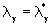actually exist, but thereappears a further symmetry, indicating the vanishing of an additional 12 of the remaining 36 components of the macroscopic energy density tensor. These are the 4´3 spacelike elements occupying the upper half of columns 5 and 6 and the left half of rows 5 and 6 of the tensor. This is to be expected on the basis of macroscopic physics. Furthermore, it has led to the following law governing the number of possible dimensions in hyperspace:

If p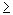0 dimensions of an Rp are empirically given, then there exists a reference space Rn containing Rp , n being an integer, such that the condition

(n1)2  1 = p (p1) (p2)

is satisfied. For p = 0, 1, and 2 the positive branch of this equation gives n = 2, while the negative branch gives n = 0. However, the latter is irrelevant for all p > 2. Finally, the equation is not satisfied for p = 3, p = 5, and all p > 6. For p = 4, on the other hand, i.e. for spacetime, n actually turns out to equal 6. Furthermore, for the material world, Rp , with p = 6, there exists a hyperspace with n = 12, containing R 6 as subspace, which in turn contains the subspace R4 . The concepts of energy and matter defined in R 4 are no longer defined in the coordinates x7 ... x12 , which again are imaginary, but the concept of volume still is. The discussion in volumes  and  is confined to a semiclassical treatment of the material world R 6 , because the nonlinear, nonHermitian R 4 relations are readily transferred to R 6 , where they become completely Hermitian.

In order to derive the smallest geometric unit mentioned above it is necessary to consider a universal background phenomenon. A suitable quantity is the general inertia of all masses, which is always equivalent to gravitational phenomena according to the principle of equivalence. A phenomenological dynamics of gravitation is derived in , which, together with a selfconsistent treatment of the massequivalent of the gravitational field energy, leads to the description of a scalar field function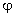by means of a nonlinear system of equations, which formally agrees with the nonlinear structural relations of R 6 . For constant x4 ,x5 , and x 6 the function(x1 ,...,x6 ) then becomes(x1 ,x2 ,x3 )of R 3 .is a real, positive gravitational potential (potential energy per unit mass), satisfying a nonlinear differential equation, which can be solved in spherical geometry and results in a transcendental algebraic equation for. The solution shows thatremains real only between the limits R and R+, where R corresponds to the Schwarzschild radius and R+ to the Hubble radius. In the range of relatively small distances (planetary systems)is almost exactly proportional to 1/r and hence practically identical to Newton¢s law of gravitation. This changes, however, in the range of very large distances, because there exists a limit,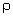, of the attractive gravitational field, lying between R and R+ ,at whichgoes to zero. This limit depends on the cube of the mean atomic weight of the field source according to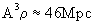. Beyondthe field becomes weakly repulsive before definitively going to zero at R+ .

A single elementary particle is characterized not only byand the limiting distances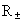of its gravitational field, but also by its Compton wavelength. R vanishes in empty space when the mass of the field source approaches zero, while R +, and the Compton wavelength all diverge. However, since the smallest geometrical unit must be a real number and a property of empty space its value has to remain finite. As shown in , only a single product having this property can be formed from the 4 characteristic lengths above. The result is an area,, bounded on all sides by geodesics, whose present size turns out to be 6.15´10 70 m 2 . This quantity, called a metron, represents the smallest area existing in empty space and requires the differential calculus to be replaced by a calculus of finite areas. Accordingly, a whole chapter in  is devoted to the development of a difference calculus considering the finite area,. This enables any differential expression to be metronized. It follows that in any subspace R n , whose dimensionality n is divisible by 2, the geometrical continuum is replaced by a metronic lattice formed by ndimensional volumes bounded on all sides by metrons. Thus, R 6 and R 12 are 6dimensional and 12dimensional metronic lattices, respectively. Since all dimensions are metronized, even time proceeds in finite, calculable steps. By the use of a difference calculus it becomes possible to introduceinto the nonlinear system of geometric structures in R 6 .

While the elementary areasare always bounded by geodesics, their area remains constant in a deformed lattice. The metronized state function then describes the projection of a deformed R 6 lattice into any Euclidian reference space, where the metrons now appear in distorted or "condensed" form, in analogy to the projection of a curved lattice onto a plane sheet, or to contour lines of constant altitude on a map providing information on the structure of a mountain range. In this respect there seems to exist a certain analogy to Regge poles. The metronic system of equations itself has the character of a selection principle, selecting out of a multiply infinite manifold of possible R 6 structures the ones whose projections into R 4 describe elementary material processes of the physical world. The operator performing this selection is called the "world selector".

Further analysis shows that the world selector separates out 4 sets of solutions, denoted by a, b, c, and d, involving 3 subspaces: A 2dimensional subspace S 2 (x 5 ,x 6 ), depending only on the two organizational coordinates, a subspace T 1 (x 4 ), describing structures in time, and a subspace R 3 (x 1 ,x 2 ,x 3 ) of physical space.

A different set of coordinates is involved in each of the 4 structures ad mentioned above: a depends on x 5 and x 6 , b on x 4 ,x 5 , and x 6 , c on x 1 ,x 2 ,x 3 ,x 5 , and x 6 , and d depends on all 6 coordinates x 1 ,...,x 6 . In every one of these combinations the coordinates are always grouped into subspaces S 2 ,T 1 , and R 3 . Note that the organizational coordinates x 5 and x 6 , constituting S 2 , appear in all elementary structures.

A sort of hermeneutics (from hermeneuo: to interpret) of the world geometry,or hermetry for short, is required for interpreting the forms a to d : a represents structures outside of R 4 , which do not in general have a physical interpretation. However, when projected into R 4 they appear as graviton fields. The world lines belonging to elements of b all lie on the twofold light cone in R 4 . For this reason they always travel with the velocity of light in R 3 and are to be interpreted as photons. In contrast to a and b, hermetry forms c and d are characterized by the inclusion of the real subspace R 3 , leading to inertia and hence to rest mass. c is interpreted as referring to neutral particles, while d refers to charged ones. In the case of d a coupling appearing between b and c characterizes the charged condition. It was possible to derive a simple relationship for an elementary charge determined by the quantum principle, whose numerical value deviates by 0.125% from the experimental electron charge.

In addition, a general mass spectrum can be derived, whose terms turn out to lie so close together that for all practical purposes they approach a continuum. This is entirely due to the fact that the spectrum is a superposition of energy terms of all hermetry forms. Thus, the practically continuous spectrum of the massless a and bterms is superimposed upon the discrete spectrum of hermetry forms c and d. For this reason a "term selector" is required for separating out the discrete mass spectra. Independently of this it is possible to derive the lower bounds of spectra c and d and to express them in terms of natural constants. They are R 3 structures representing the smallest masses, with d yielding the electron mass and c resulting in a neutral particle whose mass is about 0.1% smaller than that of the electron.

As shown in , the upper reality bound, R + , of the gravitational field increases with diminishing field source, i.e. the largest value of R, R max , results from the smallest rest mass. Thus,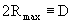is the greatest possible distance in R 3 .It is defined in  as the diameter of the universe and depends entirely on natural constants. These constants disappear if the expression foris substituted into the formula for R, and there results a higher order algebraic expression for the dependence of D on t, referred to in  as the cosmological relation. Astrophysical reasons require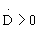, where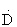. is the time derivative, which in turn results in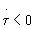. Thus, as cosmic time progresses the metronic mesh size shrinks, while the universe expands.

Going back in time, D decreases whileincreases. This ends when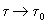encompasses a "proto universe", whose diameter, D 0 , is given by. Sincecannot become smaller, this represents an initial event in R 4 , beyond which there is no past. This instant has, therefore, been defined as the moment t = 0 of the origin of the universe. By employing an appropriate substitution D() becomes the solution of a 7th order algebraic equation. At t = 0 and at the end of time,the equation has 3 real positive, 3 real negative, and one complex solution for D. The positive solutions are interpreted as the diameters of 3 primordial spheres emerging and expanding, one after the other, after t = 0. The spheres mark the boundaries of the expanding universe, their calculated separations in time shrinking to very small values, but never to zero, as cosmic time advances. After D reaches a maximum value, contraction sets in. Finally, at the end of time the trinity of spheres, now having diameters corresponding to the 3 negative solutions, disappear one after another.

A long time after the initiation of cosmic motion at t = 0, presumably after the appearance of matter, a symmetry break of global groups leads to the development of 3 structural units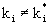, i = 1,2,3,(k i is a 6´6 tensor) in the sense of tensorial integrands of integral operators. Tensor multiplication and taking the trace according to the metronic difference calculus results in generally nonHermitian partial metric tensors g ij = Tr(k i ´ k j ) forming the elements of the full metric tensors,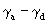. Since the 3 tensors k i are associated with the 3 primordial spheres, every elementary particle retains a memory of the cosmic origin.

The structural units k i depend on the hermetric subspaces of R 6 according to k1 (S2), k2(T1), and k3(R3). Corresponding to the 4 hermetric forms ad one can now form 4 generalized metric tensorsfrom the partial tensors g defined above. If k1(S2), k2(T1), and k3(R3) all differ from E (unit matrix) the resulting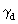(S2, T1, R3) depends on all 6 coordinates and represents hermetry form d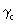(S2, R3), belonging to the spacelike hermetry form c, is obtained by putting k2 (T1) = E. In similar manner the timelike metric tensor,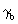(S2 ,T1 ), for hermetry form b is obtained by putting k3 (R3) = E. Finally,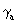(S2) for hermetry form a results from k 2 (T 1 )=k 3 (R 3 )=E.has 9 independent elements,andboth have 6, andhas 2, representing respectively 9fold, 6fold, and 2fold metrics. These polymetric structures combine to form the Hermitian metric field of the "condensor", which is also Hermitian. The condensor is an operator projecting a deformation in the 6dimensional metronic lattice of R6 into R4 , where it appears as an intricate, geometrically structured, compressed or "condensed" lattice configuration. This condensed, structured region is what we call matter constituting an elementary particle, as described in more detail below.

Thus, both the world selector and the condensor describing the internal particle structure can be split up in a manner allowing a system of partial metrics to be specified for each hermetric form. An appropriate choice of indices (ij) then results in a solution of the general energy spectrum corresponding to a separation of the discrete spectra c and d. These solutions actually yield discrete spectra of inertial masses, showing good agreement with measured particle and resonance spectra.

The following picture regarding the spectrum of elementary particles found in high energy experiments emerges from the theoretical analysis above:

Elementary particles having rest mass constitute selfcouplings of free energy. They are indeed elementary as far as their property of having rest mass is concerned, but internally they possess a very subtle, dynamic structure. For this reason they are "elementary" only in a relative sense.

Actually, such a particle appears as an elementary flow system in R6 (equivalent to energy flows) of primitive dynamic units called protosimplexes, which combine to form flux aggregates. The protosimplex flow is a circulatory, periodic motion similar to an oscillation. A particle can only exist if the flux period comprises at least one full cycle, so that the duration of a particle¢s stability is always expressible as an integer multiple of the flux period. Every dynamical R 6 structure possible constitutes a flux aggregate described by a set of 6 quantum numbers. All of them, however, result from a very simple basic symmetry, essentially determined by the configuration number k, which can only assume the values k = 1 and k = 2. The empirically introduced baryonic charge then corresponds to k  1, i.e. k = 1 refers to mesons and k = 2 to baryons.

The physically relevant parts of an R 6 flux aggregate are its k + 1 components in the physical space, R 3 , which are enveloped by a metric field. Thus, mesons contain two and baryons three components. Evidently, there exists an analogy to the empirically formulated concept of quarks. If this is true, then quarks are not fundamental particles but nonseparable, quasicorpuscular subconstituents in R 3 of a mesonic or baryonic elementary particle. In this picture the condition of quark "confinement" is unnecessary. The significance of a possible "quantum chromodynamics" will have to be derived on the basis of a unified description of possible interactions. This problem is being investigated in .

Inertial mass originates in the protosimplexes, i.e. in the basic building blocks of flux aggregates, which form the structures of the k + 1 subconstituents in R 3 . They compose 4 concentric spherical shelllike configuration zones maintaining a dynamical equilibrium, during whose existence there appears a measurable particle mass. However, an attempt to measure the mass of a subconstituent part by scattering experiments will result in a very broad, variable bandwidth of measurements, because such a mass depends on the instantaneous flux phase. The sum of the k + 1 subconstituent masses, on the other hand, is constant and gives in essence the measurable particle mass. The relevant quantity in this connection is the degree to which the 4 configuration zones in R 3 are occupied by dynamic flux elements.

For k = 1 and k = 2 there are altogether 25 sets of 6 quantum numbers each, characterizing the occupation of configuration zones and the corresponding invariant rest masses. The particles belonging to these invariant basic patterns are in turn combined into several families of spin isomorphisms, in which the spatial flux dynamics of the configuration zones is in dynamic equilibrium.

In all these terms there exists a single basic invariant framework of occupied zones, depending only on whether k = 1 or k = 2. When these occupation numbers are substituted into the mass formula derived in  this reproduces the masses of electron and proton to very good accuracy. The masses of all other ground states are produced with similar accuracy. However, the mass formula contains ratios of coupling constants, which could not at the time of writing of  be derived theoretically and had to be adjusted to fit experiments carried out at CERN in 1974. Only in  has it become possible to derive the coupling constants from first principles. A revised set of particle masses will be calculated after publication of .

It seems that the lifetime of a state depends on the deviation of its configuration zone occupation from the framework structure mentioned above. It is conceivable, in analogy to the optically active isomers of organic chemistry, that there exist isomers with spatial reflection symmetry also in the area of flux aggregates, giving rise to variations in lifetime. Perhaps the two equalmass components of the K0 meson,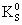and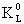, are to be interpreted in this way.

Finally, the requirement that empty space be characterized by vanishing zonal occupations and electric charge states leads to some masses in the case of k = 1, which may be interpreted as masses of neutrino states. However, these refer neither to rest masses nor to free field energies (in analogy to photons), but to quantumlike "field catalysts", i.e. masses of particles able to catalyze nuclear reactions that otherwise would not take place. They transfer group theoretical properties, arising from the sets of quantum numbers, through physical space.

The formula derived in  for the spectrum of elementary particles also depends on an integer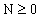, where N = 0 refers to the 25 ground state masses. For N > 0 the sets of quantum numbers again yield masses, which now denote resonance excitations of the basic structural patterns to states of higher energy. According to the dynamics of configuration zones only a single set of zonal occupations is possible for each N. Evidently, the corresponding masses represent shortlived resonance states, for all measured resonances appear among these spectra. In each case N is limited, since for every set x of quantum numbers there exists a finite resonance limit,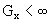, such that the closed intervals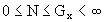0 apply to every resonance order N, including the ground state. Out of the relatively large number of logically possible particles, presentday high energy accelerator experiments only record the small subset whose probabilities of formation (depending on experimental conditions) is sufficiently large. What evidently still is lacking is a general mathematical expression relating these probabilities of formation to particle properties and experimental boundary conditions. The theory developed in  and  represents a semiclassical investigation. A third volume, entitled Strukturen der physikalischen Welt und ihrer nichtmateriellen Seite (Structures of the Physical World and its NonMaterial Aspect), , leads beyond the semiclassical domain to a dynamics in the hyperspace of R12.

Burkhard Heim
Schillerstr. 2
D37154 Northeim

 Heim, B. Elementarstrukturen der Materie, Vol. 1 (revised), Resch Verlag, Innsbruck, Austria, 1989

 Heim, B. Elementarstrukturen der Materie, Vol. 2, Resch Verlag, Innsbruck, Austria, 1984

 Dröscher, W., and Heim, B. Strukturen der physikalischen Welt und ihrer nichtmateriellen Seite, Resch Verlag, Innsbruck, Austria, 1996